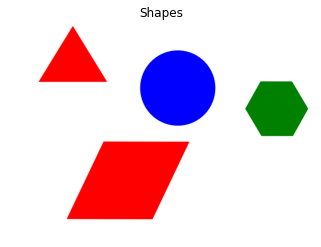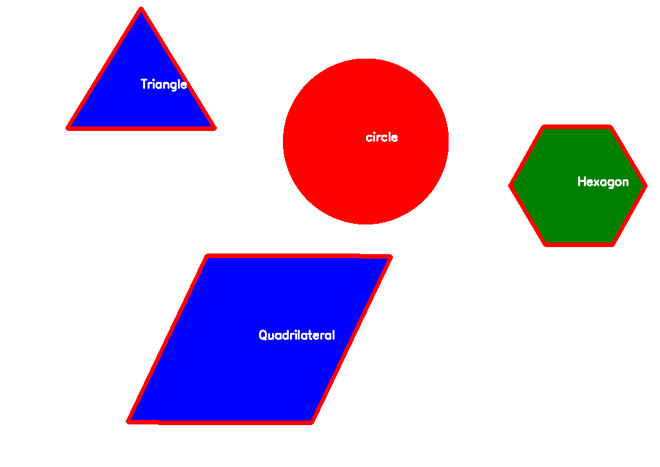# How to Detect Shapes in Images in Python using OpenCV?

• Last Updated : 13 Jan, 2021

Prerequisites: OpenCV

OpenCV is an open source library used mainly for processing images and videos to identify shapes, objects, text etc. It is mostly used with python. In this article we are going to see how to detect shapes in image. For this we need cv2.findContours() function of OpenCV, and also we are going to use cv2.drawContours() function to draw edges on images. A contour is an outline or a boundary of shape.

### Approach

• Import module
• Import image
• Convert it to grayscale image
• Apply thresholding on image and then find out contours.
• Run a loop in the range of contours and iterate through it.
• In this loop draw a outline of shapes (Using drawContours() ) and find out center point of shape.
• Classify the detected shape on the basis of a number of contour points it has and put the detected shape name at the center point of shape.

### Function Used

• cv2.findContours(): Basically this method find outs all the boundary points of shape in image.

Syntax: cv2.findContours(src, contour_retrieval, contours_approximation)

Parameters:

• src: input image n-dimensional (but for in our example we are going to use 2 dimensional image which is
mostly preferred.)
• contour_retrieval:
• cv.RETR_EXTERNAL:retrieves only the extreme outer contours
• cv.RETR_LIST:retrieves all of the contours without establishing any hierarchical relationships.
• cv.RETR_TREE:retrieves all of the contours and reconstructs a full hierarchy of nested contours.
• contours_approximation:
• cv.CHAIN_APPROX_NONE: It will store all the boundary points.
• cv.CHAIN_APPROX_SIMPLE: It will store number of end points(eg.In case of rectangle it will store 4)

Return value: list of contour points

• cv2.drawContours() : This method draws a contour. It can also draw a shape if you provide boundary points.

Syntax: cv.DrawContours(src, contour, contourIndex, colour, thickness)

Parameters:

• src: n dimensional image
• contour: contour points it can be list.
• contourIndex:
• -1:draw all the contours
• To draw individual contour we can pass here index value
• color:color values
• thickness: size of outline

Input:Program:

## Python3

 `import` `cv2``import` `numpy as np``from` `matplotlib ``import` `pyplot as plt`` ` `# reading image``img ``=` `cv2.imread(``'shapes.png'``)`` ` `# converting image into grayscale image``gray ``=` `cv2.cvtColor(img, cv2.COLOR_BGR2GRAY)`` ` `# setting threshold of gray image``_, threshold ``=` `cv2.threshold(gray, ``127``, ``255``, cv2.THRESH_BINARY)`` ` `# using a findContours() function``contours, _ ``=` `cv2.findContours(``    ``threshold, cv2.RETR_TREE, cv2.CHAIN_APPROX_SIMPLE)`` ` `i ``=` `0`` ` `# list for storing names of shapes``for` `contour ``in` `contours:`` ` `    ``# here we are ignoring first counter because ``    ``# findcontour function detects whole image as shape``    ``if` `i ``=``=` `0``:``        ``i ``=` `1``        ``continue`` ` `    ``# cv2.approxPloyDP() function to approximate the shape``    ``approx ``=` `cv2.approxPolyDP(``        ``contour, ``0.01` `*` `cv2.arcLength(contour, ``True``), ``True``)``     ` `    ``# using drawContours() function``    ``cv2.drawContours(img, [contour], ``0``, (``0``, ``0``, ``255``), ``5``)`` ` `    ``# finding center point of shape``    ``M ``=` `cv2.moments(contour)``    ``if` `M[``'m00'``] !``=` `0.0``:``        ``x ``=` `int``(M[``'m10'``]``/``M[``'m00'``])``        ``y ``=` `int``(M[``'m01'``]``/``M[``'m00'``])`` ` `    ``# putting shape name at center of each shape``    ``if` `len``(approx) ``=``=` `3``:``        ``cv2.putText(img, ``'Triangle'``, (x, y),``                    ``cv2.FONT_HERSHEY_SIMPLEX, ``0.6``, (``255``, ``255``, ``255``), ``2``)`` ` `    ``elif` `len``(approx) ``=``=` `4``:``        ``cv2.putText(img, ``'Quadrilateral'``, (x, y),``                    ``cv2.FONT_HERSHEY_SIMPLEX, ``0.6``, (``255``, ``255``, ``255``), ``2``)`` ` `    ``elif` `len``(approx) ``=``=` `5``:``        ``cv2.putText(img, ``'Pentagon'``, (x, y),``                    ``cv2.FONT_HERSHEY_SIMPLEX, ``0.6``, (``255``, ``255``, ``255``), ``2``)`` ` `    ``elif` `len``(approx) ``=``=` `6``:``        ``cv2.putText(img, ``'Hexagon'``, (x, y),``                    ``cv2.FONT_HERSHEY_SIMPLEX, ``0.6``, (``255``, ``255``, ``255``), ``2``)`` ` `    ``else``:``        ``cv2.putText(img, ``'circle'``, (x, y),``                    ``cv2.FONT_HERSHEY_SIMPLEX, ``0.6``, (``255``, ``255``, ``255``), ``2``)`` ` `# displaying the image after drawing contours``cv2.imshow(``'shapes'``, img)`` ` `cv2.waitKey(``0``)``cv2.destroyAllWindows()`

Output:My Personal Notes arrow_drop_up